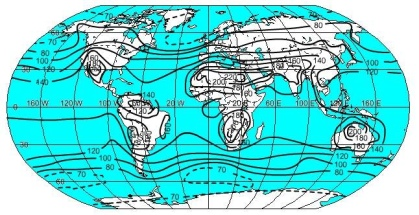# 4.4: Global Patterns of Insolation, Net radiation, and Heat

$$\newcommand{\vecs}{\overset { \rightharpoonup} {\mathbf{#1}} }$$ $$\newcommand{\vecd}{\overset{-\!-\!\rightharpoonup}{\vphantom{a}\smash {#1}}}$$$$\newcommand{\id}{\mathrm{id}}$$ $$\newcommand{\Span}{\mathrm{span}}$$ $$\newcommand{\kernel}{\mathrm{null}\,}$$ $$\newcommand{\range}{\mathrm{range}\,}$$ $$\newcommand{\RealPart}{\mathrm{Re}}$$ $$\newcommand{\ImaginaryPart}{\mathrm{Im}}$$ $$\newcommand{\Argument}{\mathrm{Arg}}$$ $$\newcommand{\norm}{\| #1 \|}$$ $$\newcommand{\inner}{\langle #1, #2 \rangle}$$ $$\newcommand{\Span}{\mathrm{span}}$$ $$\newcommand{\id}{\mathrm{id}}$$ $$\newcommand{\Span}{\mathrm{span}}$$ $$\newcommand{\kernel}{\mathrm{null}\,}$$ $$\newcommand{\range}{\mathrm{range}\,}$$ $$\newcommand{\RealPart}{\mathrm{Re}}$$ $$\newcommand{\ImaginaryPart}{\mathrm{Im}}$$ $$\newcommand{\Argument}{\mathrm{Arg}}$$ $$\newcommand{\norm}{\| #1 \|}$$ $$\newcommand{\inner}{\langle #1, #2 \rangle}$$ $$\newcommand{\Span}{\mathrm{span}}$$$$\newcommand{\AA}{\unicode[.8,0]{x212B}}$$

Patterns of insolation and net radiation which determine the location of plants, animals, climate, soils and other elements of our physical environment can be discerned from Figures $$\PageIndex{1}$$ through $$\PageIndex{3}$$. Figure $$\PageIndex{1}$$ illustrates the latitudinal distribution of incoming solar radiation and outgoing terrestrial radiation. From approximately 35o N to 35o S latitude (the red area of the graph) there is a surplus of energy as incoming radiation exceeds outgoing. The blue regions indicate that there is more outgoing energy than incoming, yielding a net loss of energy from the Earth's surface. One might ask then why the middle to higher latitudes aren't getting colder through time as a result of the net loss, and the subtropical to equatorial regions getting constantly hotter due to the net gain. The reason is that the energy is redistributed by circulation of the atmosphere and oceans. Heat gained in the tropics is transported poleward by the global circulation of air and warm ocean currents to heat higher latitude regions. Cooler air from the higher latitudes and cold ocean currents push equatorward to cool the lower latitudes. This process of redistributing energy in the Earth system helps maintain a long-term energy balance.Figure $$\PageIndex{1}$$: Latitudinal Variation of the Radiation Balance

## Insolation

Figure $$\PageIndex{1}$$ simplifies the geographic distribution of insolation. For the Earth as a whole, particular patterns can be accounted for by variation in surface features that impact insolation. Insolation maxima are found in the tropical and subtropical deserts of the earth. Here, high sun angles and a lack of cloud cover in desert regions allow much solar radiation to the surface. Insolation decreases to a minimum at the poles where low sun angles and the fact that the Sun doesn't rise above the horizon nearly half the year reduces annual insolation.Figure $$\PageIndex{2}$$: Annual global distribution of solar radiation (Kcal/cm2) (after Sellers, W. D., 1965)Figure $$\PageIndex{3}$$: Annual global distribution of net radiation (Kcal/cm2) (after Sellers, W. D., 1965)